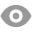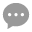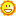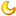# 两阳夹一阴形态测试和代码汇总89 |5 |王博 | 2020-4-17 15:47:39 | 显示全部楼层 |阅读模式
 目 录 2楼 测试一【两阳夹一阴】 3楼 测试二【两阳夹一阴+出现在上升趋势中】 4楼 测试三【两阳夹一阴+出现在下跌趋势中】 5楼 测试四【两阳夹一阴+收盘价未突破形态高点】 6楼 测试五【两阳夹一阴+收盘价突破形态高点】

 测试一代码【两阳夹一阴】 1) 测试买卖条件买入条件：形成两阳夹一阴形态，以收盘价买入卖出条件：第二天收盘价，卖出 2）测试代码//定义仓位FUND:=10000000;LOTS:=INTPART(FUND*0.8/(CLOSE+FEE));//计算手数，使每次开仓金额都是800W //定义K线KXST:=ABS(O-C);//K线实体长度YXCD:=H-L;//K线影线长度BT:=KXST>YXCD*0.5;//棒体实体 //定义两阳夹一阴DFP1:=REF(ISUP,2) AND REF(BT,2);//第一根为阳棒体DFP2:=REF(ISDOWN,1) AND REF(BT,1);//第二根为阴棒体DFP3:=ISUP AND BT;//第三根为阳棒体DFP:=DFP1 AND DFP2 AND DFP3; DFP,BK(LOTS);//出现两阳夹一阴，以收盘价买入BARSBK=1,SP(BKVOL);//第二天，以收盘价卖出

 测试二代码【两阳夹一阴+出现在上升趋势中】1）测试买卖条件买入条件：形成两阳夹一阴，并且第1根K线收盘价大于当日的20日均线，买入卖出条件：第二天收盘价，卖出 2）测试代码 //定义仓位 FUND:=10000000; LOTS:=INTPART(FUND*0.8/(CLOSE+FEE));//计算手数，使每次开仓金额都是800W //定义均线 MA20:MA(C,20);//20日均线 //定义K线 KXST:=ABS(O-C);//K线实体长度 YXCD:=H-L;//K线影线长度 BT:=KXST>YXCD*0.5;//棒体实体 //定义两阳夹一阴 DFP1:=REF(ISUP,2) AND REF(BT,2);//第一根为阳棒体 DFP2:=REF(ISDOWN,1) AND REF(BT,1);//第二根为阴棒体 DFP3:=ISUP AND BT;//第三根为阳棒体 DFP4:=REF(C,2)>REF(MA20,2);//上升趋势 DFP:=DFP1 AND DFP2 AND DFP3 AND DFP4; DFP,BK(LOTS);//两阳夹一阴+出现在上升趋势中 BARSBK=1,SP(BKVOL);//第二天，以收盘价卖出

 测试三代码【两阳夹一阴+出现在下跌趋势中】1）测试买卖条件买入条件：形成两阳夹一阴，并且第1根K线收盘价小于当日的20日均线，买入卖出条件：第二天收盘价，卖出 2）测试代码 //定义仓位 FUND:=10000000; LOTS:=INTPART(FUND*0.8/(CLOSE+FEE));//计算手数，使每次开仓金额都是800W //定义均线 MA20:MA(C,20);//20日均线 //定义K线 KXST:=ABS(O-C);//K线实体长度 YXCD:=H-L;//K线影线长度 BT:=KXST>YXCD*0.5;//棒体实体 //定义两阳夹一阴 DFP1:=REF(ISUP,2) AND REF(BT,2);//第一根为阳棒体 DFP2:=REF(ISDOWN,1) AND REF(BT,1);//第二根为阴棒体 DFP3:=ISUP AND BT;//第三根为阳棒体 DFP4:=REF(C,2)

 测试四代码【两阳夹一阴+收盘价未突破形态高点】1）测试买卖条件买入条件：形成两阳夹一阴，并且第3根K线收盘价小于前2天高点，买入卖出条件：第二天收盘价，卖出 2）测试代码 //定义仓位 FUND:=10000000; LOTS:=INTPART(FUND*0.8/(CLOSE+FEE));//计算手数，使每次开仓金额都是800W //定义K线 KXST:=ABS(O-C);//K线实体长度 YXCD:=H-L;//K线影线长度 BT:=KXST>YXCD*0.5;//棒体实体 //定义两阳夹一阴 DFP1:=REF(ISUP,2) AND REF(BT,2);//第一根为阳棒体 DFP2:=REF(ISDOWN,1) AND REF(BT,1);//第二根为阴棒体 DFP3:=ISUP AND BT;//第三根为阳棒体 DFP4:=C

 测试五代码【两阳夹一阴+收盘价突破形态高点】1）测试买卖条件买入条件：形成两阳夹一阴，并且第3根K线收盘价大于前两天高点，买入卖出条件：第二天收盘价，卖出 2）测试代码 //定义仓位 FUND:=10000000; LOTS:=INTPART(FUND*0.8/(CLOSE+FEE));//计算手数，使每次开仓金额都是800W //定义K线 KXST:=ABS(O-C);//K线实体长度 YXCD:=H-L;//K线影线长度 BT:=KXST>YXCD*0.5;//棒体实体 //定义两阳夹一阴 DFP1:=REF(ISUP,2) AND REF(BT,2);//第一根为阳棒体 DFP2:=REF(ISDOWN,1) AND REF(BT,1);//第二根为阴棒体 DFP3:=ISUP AND BT;//第三根为阳棒体 DFP4:=C>HV(H,2);//收盘价突破形态最高价 DFP:=DFP1 AND DFP2 AND DFP3 AND DFP4; DFP,BK(LOTS);//两阳夹一阴+收盘价突破形态高点，买入 BARSBK=1,SP(BKVOL);//第二天，卖出

 您需要登录后才可以回帖 登录 | 立即注册 本版积分规则 回帖后跳转到最后一页79主题 1081积分1081